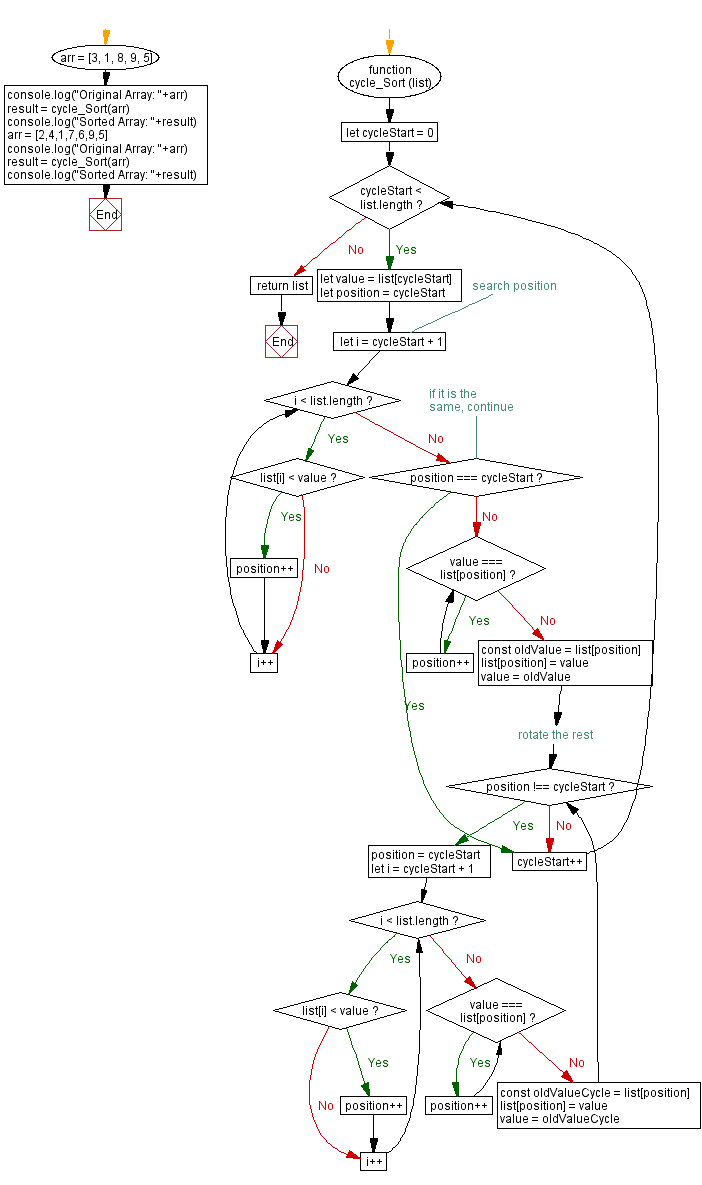# JavaScript Sorting Algorithm: Cycle sort

## JavaScript Sorting Algorithm: Exercise-28 with Solution

From Wikipedia,
Cycle sort is an in-place, unstable sorting algorithm, a comparison sort that is theoretically optimal in terms of the total number of writes to the original array, unlike any other in-place sorting algorithm. It is based on the idea that the permutation to be sorted can be factored into cycles, which can individually be rotated to give a sorted result. Unlike nearly every other sort, items are never written elsewhere in the array simply to push them out of the way of the action. Each value is either written zero times, if it's already in its correct position, or written one time to its correct position. This matches the minimal number of overwrites required for a completed in-place sort.

Write a JavaScript program to sort a list of elements using the Cycle sort algorithm.

Sample Data: Original Array: [3, 1, 8, 9, 5]
Sorted Array: [1, 3, 5, 8, 9]
Original Array: [2, 4, 1, 7, 6, 9, 5]
Sorted Array: [1, 2, 4, 5, 6, 7, 9]

Sample Solution:

JavaScript Code:

``````/**
* cycleSort takes an input array of numbers and returns the array sorted in increasing order.
*
* @param {number[]} list An array of numbers to be sorted.
* @return {number[]} An array of numbers sorted in increasing order.
*/

function cycle_Sort (list) {
for (let cycleStart = 0; cycleStart < list.length; cycleStart++) {
let value = list[cycleStart]
let position = cycleStart

// search position
for (let i = cycleStart + 1; i < list.length; i++) {
if (list[i] < value) {
position++
}
}
// if it is the same, continue
if (position === cycleStart) {
continue
}
while (value === list[position]) {
position++
}

const oldValue = list[position]
list[position] = value
value = oldValue

// rotate the rest
while (position !== cycleStart) {
position = cycleStart
for (let i = cycleStart + 1; i < list.length; i++) {
if (list[i] < value) {
position++
}
}
while (value === list[position]) {
position++
}
const oldValueCycle = list[position]
list[position] = value
value = oldValueCycle
}
}
return list
}

arr = [3, 1, 8, 9, 5]
console.log("Original Array: "+arr)
result = cycle_Sort(arr)
console.log("Sorted Array: "+result)
arr = [2,4,1,7,6,9,5]
console.log("Original Array: "+arr)
result = cycle_Sort(arr)
console.log("Sorted Array: "+result)
```
```

Sample Output:

```"Original Array: 3,1,8,9,5"
"Sorted Array: 1,3,5,8,9"
"Original Array: 2,4,1,7,6,9,5"
"Sorted Array: 1,2,4,5,6,7,9"
"Original Array: 3,1,8,9,5"
"Sorted Array: 1,3,5,8,9"
"Original Array: 2,4,1,7,6,9,5"
"Sorted Array: 1,2,4,5,6,7,9"
```

Flowchart:Live Demo:

See the Pen searching-and-sorting-algorithm-exercise-28 by w3resource (@w3resource) on CodePen.

Improve this sample solution and post your code through Disqus

Previous: Binary Insertion sort.
Next: Odd - Even sort.

What is the difficulty level of this exercise?

Test your Programming skills with w3resource's quiz.

﻿